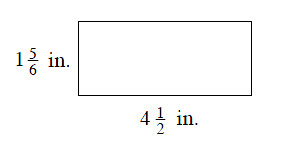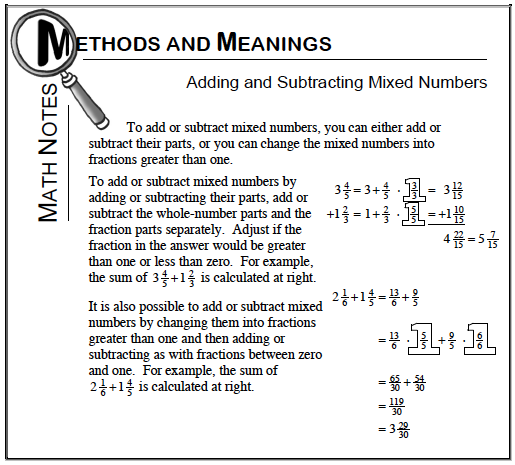### Home > MC1 > Chapter 9 > Lesson 9.2.5 > Problem9-108

9-108.

Find the area and perimeter of the rectangle at right. Show your work.Remember, the area is the number of one unit squares that fit inside of a shape.
The area of a rectangle is also equal to the length times the width.
Can you calculate the area of this figure? Your answer should be in inches2.

To multiply mixed numbers, you can convert them to fractions greater than one, and then multiply.

Also, the perimeter of a shape is the distance around it. Can you find the sum of the rectangle's four sides?

For help adding mixed numbers, refer to the Math Notes box below.The area of the rectangle is $8\frac{1}{4}$ square inches.

Did you find the rectangle's perimeter? Don't forget to show your work!